# PSEB 11th Class Maths Solutions Chapter 8 Binomial Theorem Miscellaneous Exercise

Punjab State Board PSEB 11th Class Maths Book Solutions Chapter 8 Binomial Theorem Miscellaneous Exercise Questions and Answers.

## PSEB Solutions for Class 11 Maths Chapter 8 Binomial Theorem Miscellaneous Exercise

Question 1.
Find a, b and n in the expansion of (a + b)n if the first three terms of the expansion are 729, 7290 and 30375, respectively.
It is known that (r + 1)th term, (Tr + 1), in the binomial expansion of (a + b)n is given by Tr + 1 = $${ }^{n} C_{r}$$ an – r r= a
The first three terms of the expansion are given as 729, 7290, and 30375 respectively.
Therefore, we obtain
T1 = $${ }^{2} C_{0} a^{n-0} b^{0}$$
= an = 729 ……………..(i)

T2 = $${ }^{n} C_{1} a^{n-1} b^{1}$$
= n an – 1 b = 7290 …………….(ii)

T3 = $${ }^{n} C_{2} a^{n-2} b^{2}$$
= $$\frac{n(n-1)}{2} a^{n-2} b^{2}$$ = 30375 ……………….(iii)

Dividing eq. (ii) by eq. (i), we obtain $$\frac{n a^{n-1} b}{a^{n}}=\frac{7290}{729}$$
⇒ $$\frac{n b}{a}$$ = 10 ……………….(iv)

Dividing eq. (iii) by eq. (ii), we obtain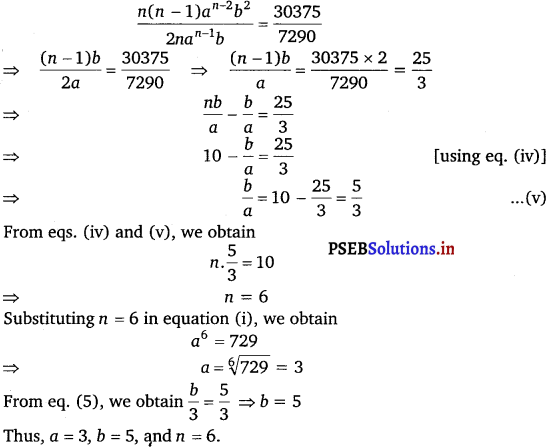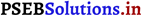Question 2.
Find a if the coefficient of x2 and x3 in the expansion of (3 + ax)9 are equal.
It is known that (r + 1)th term, (Tr + 1), in the binomial expansion of (a + b)n is given by Tr + 1 = $${ }^{n} C_{r} a^{n-r} b^{r}$$

Assuming that x2 occurs in the (r + 1)th term in the expansion of (3 + ax)9, we obtain
Tr + 1 = $${ }^{9} C_{r}(3)^{9-r}(a x)^{r}$$
= $${ }^{9} C_{r}(3){ }^{9-r} a^{r} x^{r}$$

Comparing the indices of x in x2 and in Tr + 1 we obtain r = 2
Thus, the coefficient of x2 is
$${ }^{9} C_{2}(3)^{9-2} a^{2}=\frac{9 !}{2 ! 7 !}(3)^{7} a^{2}=36(3)^{7} a^{2}$$

Assuming that x3 occurs in the (k + 1)th term in the expansion of (3 + ax)9, we obtain
Tk + 1 = $${ }^{9} C_{k}(3)^{9-k}(a x)^{k}={ }^{9} C_{k}(3)^{9-k} a^{k} x^{k}$$

Comparing the indices of x in x3 in Tr + 1, we obtain k = 3
Thus, the coefficient of x3 is
$${ }^{9} C_{3}(3)^{9-3} a^{3}=\frac{9 !}{3 ! 6 !}(3)^{6} a^{3}=84(3)^{6} a^{3}$$

It is given that the coefficients of x2 and x3 are same.
84 (3)6 a3 = 36 (3)7 a2
⇒ 84a = 36 × 3
⇒ a = $$\frac{36 \times 3}{84}=\frac{108}{84}$$
⇒ a = $$\frac{9}{7}$$

Thus, the required value of a is $$\frac{9}{7}$$.Question 3.
Find the coefficient of x5 in the product (1 + 2x)6(1 – x)7 using binomial theorem.
Using Binomial Theorem, the expressions, (1 + 2x)6 and (1 – x)7, can be expanded as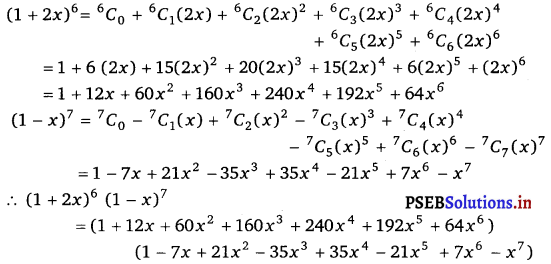The complete multiplication of the two brackets is not required to be carried out.
Only those terms, which involve x5, are required.
The terms containing x5 are
1 (- 21x5) + (12x) (35x4) + (60x2) (- 35x3) + (160x3) (21x2) + (240x4) (- 7x) + (192 x5)(1)
= 171 x5
Thus, the coefficient of x5 in the given product.
= – 21 + 420 – 2100 + 3360 -1 680 +192 = 171.Question 4.
If a and b are distinct integers, prove that a – b is a factor of an – bn, whenever n is a positive integer.
[Hint : write an = (a – b + b)n and expand]
In order to prove that (a – b) is a factor of (an – bn), it has to be proved that
an – bn = k(a – b), where k is some natural number
It can be written that, a = a – b + b
∴ an = (a – b + b)n = [(a – b) + b]n
= $${ }^{n} C_{0}(a-b)^{n}+{ }^{n} C_{1}(a-b){ }^{n-1} b+\ldots+{ }^{n} C_{n-1}(a-b) b^{n-1}+{ }^{n} C_{n} b^{n}$$

= $$(a-b)^{n}+{ }^{n} C_{1}(a-b)^{n-1} b+\ldots+{ }^{n} C_{n-1}(a-b) b^{n-1}+b^{n}$$

$$a^{n}-b^{n}=(a-b)\left[(a-b)^{n-1}+{ }^{n} C_{1}(a-b)^{n-2} b+\ldots+{ }^{n} C_{n-1} b^{n-1}\right]$$

an – bn = k (a -b)

where k = $$\left[(a-b)^{n-1}+{ }^{n} C_{1}(a-b)^{n-2} b+\ldots+{ }^{n} C_{n-1} b^{n-1}\right]$$ is a natural number
This shows that (a – b) is a factor of (an – bn), where n is a positive integer.Question 5.
Evaluate (√3 + √2)6 – (√3 – √2)6.
Firstly, the expression (a + b)6 – (a – b)6 is simplified by using Binomial Theorem.
This can be done as
(a + b)6 = $${ }^{6} C_{0}$$ a6 + $${ }^{6} C_{1}$$ a5 b + $${ }^{6} C_{2}$$ a4 b2 + $${ }^{6} C_{3}$$ a3b3 + $${ }^{6} C_{4}$$ a2b4 + $${ }^{6} C_{5}$$ ab5 + $${ }^{6} C_{6}$$ b6

= a6 + 6 a5b + 15 a4b2 + 20 a3b3 + 15 a2b4 + 6 ab5 + b6 (a – b)6

= $${ }^{6} C_{0}$$ a6 – $${ }^{6} C_{1}$$ a5b + $${ }^{6} C_{2}$$ a4b2 – $${ }^{6} C_{3}$$ a3b3 + $${ }^{6} C_{4}$$ a2b4 – $${ }^{6} C_{5}$$ a1b5 + $${ }^{6} C_{6}$$ b6

= a6 – 6 a5b + 15 a4b2 – 20 a3b3 + 15 a2b4 – 6ab5 + b6

.-. (a + b)6 – (a – b)6 = 2[6a5b + 20a3b3 + 6ab5]

Putting a = √3 and b = √2, we obtain (√3 + √2)6 – (√3 – √2)6
= 2[6(√3)5 (√2) + 20 (√3)3 (√2)3 + 6 (√3) (√2)5]
= 2[54 √6 +120 √6 + 24 √6]
= 2 × 198 √6 = 396√6.Question 6.
Find the value of (a2 + $$\sqrt{a^{2}-1}$$)4 + (a2 – $$\sqrt{a^{2}-1}$$)4.
Firstly, the expression (x + y)4 + (x – y)4 is simplified by using Binomial Theorem.
This can be done as
(x + y)4 = $${ }^{4} C_{0}$$ x4 + $${ }^{4} C_{1}$$ x3y + $${ }^{4} C_{2}$$ x2y2 + $${ }^{4} C_{3}$$ xy3 + $${ }^{4} C_{4}$$ y4
= x4 + 4x3y + 6x2y2 + 4xy3 + y4

(x – y)4 = $${ }^{4} C_{0}$$ x4 – $${ }^{4} C_{1}$$ x3y + $${ }^{4} C_{2}$$ x2y2 – $${ }^{4} C_{3}$$ xy3 + $${ }^{4} C_{4}$$ y4
= x4 – 4x3y + 6x2y2 – 4xy3 + y4

∴ (x + y)4 + (x – y)4 = 2(x4 + 6x2y2 + y4).

Putting x = a2 and y = $$\sqrt{a^{2}-1}$$, we obtain
(a2 + $$\sqrt{a^{2}-1}$$)4 + (a2 – $$\sqrt{a^{2}-1}$$)4
= 2 [(a2)4 + 6(a2)2 (Ja2 -l)2 + $$\left(\sqrt{a^{2}-1}\right)$$4]
= 2 [a8 + 6a4 (a2 – 1) + (a2 – 1)2]
= 2 [a8 + 6a6 – 6a4 + a4 – 2a2 + 1]
= 2 [a8 + 6a6 – 5a4 – 2a2 + 1]
= 2a8 + 12a6 – 10a4 – 4a2 + 2.Question 7.
Find an approximation of (0.99)5 using the first three terms of its expansion.
We have, (0.99)5 = (1 – 0.01)5
= 1 – $${ }^{5} C_{1}$$ × (0.01) + $${ }^{5} C_{2}$$ × (0.01)2 – ……….
= 1 – 0.05 + 10 × 0.0001 – ………..
= 1.001 – 0.05 = 0.951.

Question 8.
Find n, if the ratio of the fifth term from the beginning to the fifth term from the end in the expression of $$\left(\sqrt{2}+\frac{1}{\sqrt{3}}\right)^{n}$$ is √6 : 1.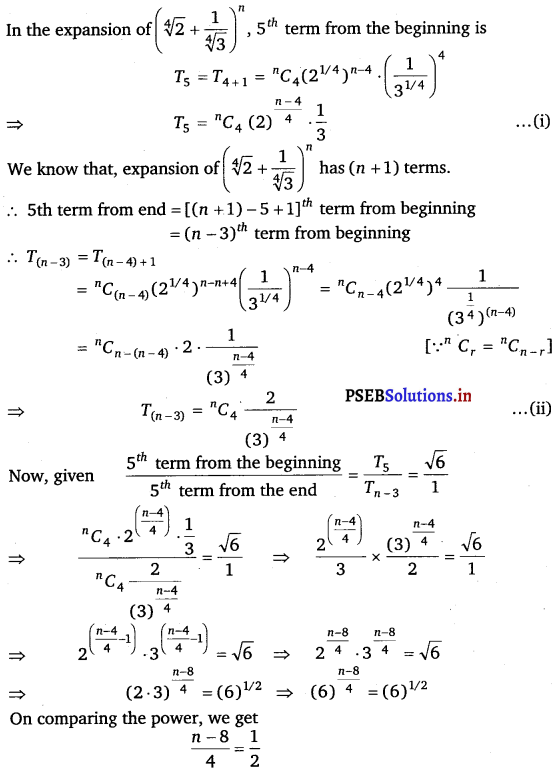2n – 16 = 4
⇒ 2n = 20
⇒ n = $$\frac{20}{2}$$
⇒ n = 10.Question 9.
Expand using Binomial Theorem $$\left(1+\frac{x}{2}-\frac{2}{x}\right)^{4}$$, x ≠ 0.
Using Binomial Theorem, the given expression $$\left(1+\frac{x}{2}-\frac{2}{x}\right)^{4}$$ can be expanded as $$\left[\left(1+\frac{x}{2}\right)-\frac{2}{x}\right]^{4}$$,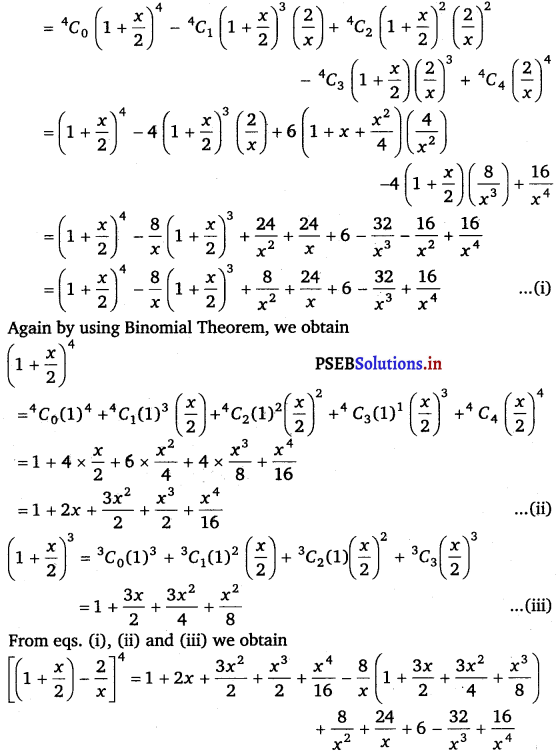= $$1+2 x+\frac{3}{2} x^{2}+\frac{x^{3}}{2}+\frac{x^{4}}{16}-\frac{8}{x}-12-6 x$$ – $$x^{2}+\frac{8}{x^{2}}+\frac{24}{x}+6-\frac{32}{x^{3}}+\frac{16}{x^{4}}$$

= $$\frac{16}{x}+\frac{8}{x^{2}}-\frac{32}{x^{3}}+\frac{16}{x^{4}}-4 x+\frac{x^{2}}{2}+\frac{x^{3}}{2}+\frac{x^{4}}{16}-5$$.Question 10.
Find the expansion of (3x2 – 2ax + 3a2)3 using binomial theorem.
Using Binomial Theorem, the given expression (3x2 – 2ax + 3a2)3 can be expanded as [(3x2 – 2ax) + 3a2]3

= $${ }^{3} C_{0}$$ (3x2 – 2ax)3 + $${ }^{3} C_{1}$$ (3x2 – 2ax)2 (3a2) + $${ }^{3} C_{2}$$ (3x2 – 2ax) (3a2)2 + $${ }^{3} C_{3}$$ (3a2)3

= (3x2 – 2ax)3 + 3 (9x4 – 12ax3 + 4a2x2) (3a2) + 3 (3x2 – 2ax) (9a4) + 27a6

= (3x2 – 2ax)3 + 81a2x4 – 108a3x3 + 36a4x2 + 81a4x2 – 54a5x + 27a6

= (3x2 -2ax)3 + 81 a2x4 – 108a3x3 + 117a4x2 – 54a5x + 27a6 …………….(i)

Again by using Binomial Theorem, we obtain (3x2 – 2ax)3
= $${ }^{3} C_{0}$$ (3x2)3 – $${ }^{3} C_{1}$$ (3x2)2 (2ax) + $${ }^{3} C_{2}$$ (3x2) (2ax)2 – $${ }^{3} C_{3}$$(2ax)3
= 27x6 – 3(9x4) (2ax) + 3(3x2) (4a2x2) – 8a3x3
= 27x6 – 54ax5 + 36a2x4 – 8a3x3 ………….(ii)

From eqs. (i) and (ii), we obtain (3x2 – 2ax + 3a2)3
= 27x6 – 54ax5 + 36a2 x4 – 8a3x3 + 81a2x4 – 108a3x3 + 117a4x2 – 54a5x + 27a6
= 27x6 – 54ax5 + 117a2x4 – 116a3x3 + 117a4x2 – 54a5x + 27a6.